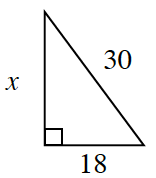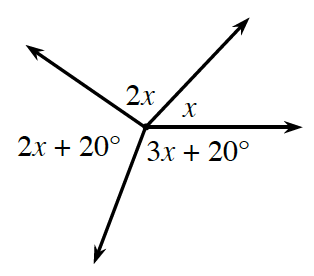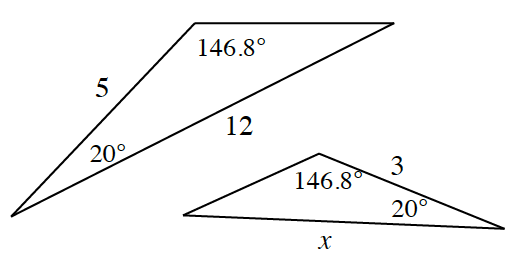### Home > CCG > Chapter 4 > Lesson 4.1.4 > Problem4-40

4-40.

Use the relationships in the diagrams below to write an equation and solve for $x$.

1.This is a right triangle, so use the Pythagorean Theorem to solve for $x$.

1.What is the sum of these angles?
How can you use the sum of the angles to write an equation and solve for $x$?

1.Are these triangles similar?
If so, how can you use their similarity to write an equation and solve for $x$?